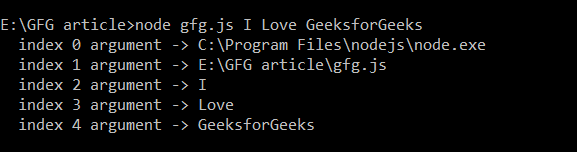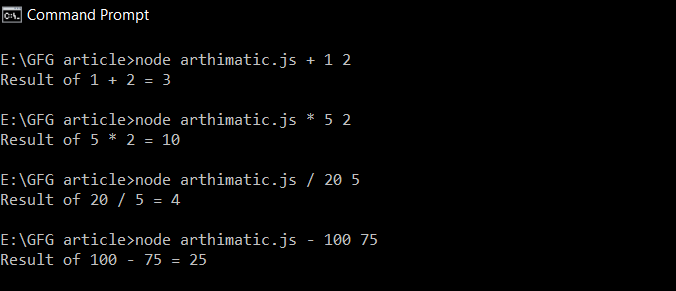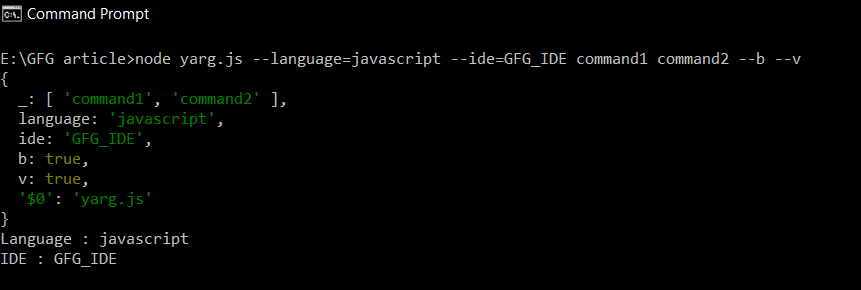# How to parse command line arguments in node.js ?

• Difficulty Level : Medium
• Last Updated : 10 Dec, 2020

Command-line arguments are strings of text used to pass additional information to a program when an application is run through the command-line interface (CLI) of an operating system. In Node.js, all command-line arguments received by the shell are given to the process in an array called argv(arguments-values).

We will use two methods to parse command-line arguments via process.argv array as well as popular package yargs

Method 1: Using process.argv: It is the simplest way to receive arguments with the help of process.argv array. Node.js exposes this array for every running process in the form of process.argv. The first element of process.argv array is the file system path pointing to Node executable file. The second element is the path of the JavaScript file that is being executed and the rest array elements are the arguments passed via cmd.

Note: The first two elements of process.argv array are always present even if we don’t pass any arguments.

Example 1: Filename: gfg.js

## Javascript

 `for``(let i = 0; i < process.argv.length; ++i) {``    ``console.log(`index \${i} argument -> \${process.argv[i]}`);``}`

Run the gfg.js file using the following command by passing arguments:

`node gfg.js I Love GeeksforGeeks`

Output:Using process.argv

Example 2: Program to perform an arithmetic operation according to the arguments passed via cmd.

Filename: arthimatic.js

## Javascript

 `// To trim first 2 elements``const arg = process.argv.slice(2);`` ` `arg = Number(arg);``arg = Number(arg);`` ` `switch` `(arg) {``    ``case` `'+'``:``        ``console.log(`Result of \${arg} ``        ``+ \${arg} = \${arg + arg}`);``        ``break``;`` ` `    ``case` `'*'``:``        ``console.log(`Result of \${arg} ``        ``* \${arg} = \${arg * arg}`);``        ``break``;`` ` `    ``case` `'-'``:``        ``console.log(`Result of \${arg} ``        ``- \${arg} = \${arg - arg}`);``        ``break``;`` ` `    ``case` `'/'``:``        ``if` `(arg == 0) {``            ``console.log(``                ``'cannot be divided by zero!!'``);``        ``} ``else` `{``            ``console.log(`Result of \${arg} ``            ``/ \${arg} = \${arg / arg}`);``        ``}``        ``break``;`` ` `    ``case` `'%'``:``        ``if` `(arg == 0) {``            ``console.log(``                ``'cannot be divided by zero!!'``);``        ``} ``else` `{``            ``console.log(`Result of \${arg} ``            ``% \${arg} = \${arg % arg}`);``        ``}``        ``break``;`` ` `    ``default``: console.log(``        ```operation cannot be performed!!`);``}`

Run the arthimatic.js file by passing the following arguments:

Output:Program to perform the arithmetic operation according to the arguments passed via cmd.

Method 2: Using yargs module: Passing arguments via cmd becomes tedious when we start working with flags or if your server needed a lot of arguments.

`app -h host -p port -r -v -b --quiet -x -o outfile`

To solve this, we can use the third library module such as yargs to parse the arguments passed via cmd. In this module, you can pass arguments as a key-value pair and later access them with the help of a key. The .argv gets the arguments as a plain old object.

Install yargs module using the following command:

`npm install yargs --save`

Example: Filename: yarg.js

## Javascript

 `const args = require(``'yargs'``).argv;``console.log(args);``console.log(`Language : \${args.language}`);``console.log(`IDE : \${args.ide}`);`

To run the file, execute the following command:

node yarg.js –language=javascript –ide=GFG_IDE command1 command2 –b –v

Output:Using yargs module

Note:

• argv.\$0 contains the name of the script file which is to execute.
• argv._ is an array containing each element not attached to an option(or flag) these elements are referred to as commands in yargs.
• The flags such as argv.time, argv.b, etc become the property of the argv. The flags must be passed as –flag. Example: node app.js –b

My Personal Notes arrow_drop_up